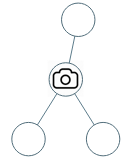Formatted question description: https://leetcode.ca/all/968.html

# 968. Binary Tree Cameras (Hard)

Given a binary tree, we install cameras on the nodes of the tree.

Each camera at a node can monitor its parent, itself, and its immediate children.

Calculate the minimum number of cameras needed to monitor all nodes of the tree.

Example 1:Input: [0,0,null,0,0]
Output: 1
Explanation: One camera is enough to monitor all nodes if placed as shown.


Example 2:Input: [0,0,null,0,null,0,null,null,0]
Output: 2
Explanation: At least two cameras are needed to monitor all nodes of the tree. The above image shows one of the valid configurations of camera placement.


Note:

1. The number of nodes in the given tree will be in the range [1, 1000].
2. Every node has value 0.

Companies:

Related Topics:
Dynamic Programming, Tree, Depth-first Search

## Solution 1.

Assumption: we can alter the value of the tree node.

Use postorder traversal. Let val convey the state of the node:

• 0 means uncovered.
• 1 means covered
• 2 means having camera

If node is NULL, we regard it as 1.

• If either of my left/right child is uncovered, I have to put a camera.
• Otherwise, if either of my left/right child has camera, I’m covered and skip me.
• Otherwise (both children are covered), if I’m root, I have to put a camera.
• Otherwise, skip me.
// OJ: https://leetcode.com/problems/binary-tree-cameras/

// Time: O(N)
// Space: O(logN)
class Solution {
private:
TreeNode *R;
int postorder(TreeNode* root) {
if (!root) return 0;
int ans = postorder(root->left) + postorder(root->right);
int left = root->left ? root->left->val : 1;
int right = root->right ? root->right->val : 1;
if (left == 0 || right == 0) {
root->val = 2;
return ans + 1;
} else if (left == 2 || right == 2) {
root->val = 1;
return ans;
} else return root == R ? ans + 1 : ans;
}
public:
int minCameraCover(TreeNode* root) {
R = root;
return postorder(root);
}
};


## Solution 2.

If we can’t have the assumption in Solution 1, we can use the return value to return my state to my parent.

// OJ: https://leetcode.com/problems/binary-tree-cameras/

// Time: O(N)
// Space: O(logN)
class Solution {
private:
int ans = 0;
int postorder(TreeNode *root) {
if (!root) return 1;
int left = postorder(root->left);
int right = postorder(root->right);
if (left == 0 || right == 0) {
++ans;
return 2;
} else return left == 2 || right == 2 ? 1 : 0;
}
public:
int minCameraCover(TreeNode* root) {
return postorder(root) == 0 ? ans + 1 : ans;
}
};


Java

/**
* Definition for a binary tree node.
* public class TreeNode {
*     int val;
*     TreeNode left;
*     TreeNode right;
*     TreeNode(int x) { val = x; }
* }
*/
class Solution {
public int minCameraCover(TreeNode root) {
if (root == null)
return 0;
List<TreeNode> list = new ArrayList<TreeNode>();
queue.offer(root);
while (!queue.isEmpty()) {
TreeNode node = queue.poll();
TreeNode left = node.left, right = node.right;
if (left != null)
queue.offer(left);
if (right != null)
queue.offer(right);
}
Map<TreeNode, int[]> map = new HashMap<TreeNode, int[]>();
for (int i = list.size() - 1; i >= 0; i--) {
TreeNode node = list.get(i);
if (node.left == null && node.right == null)
map.put(node, new int[]{0, Integer.MAX_VALUE / 10, 1});
else if (node.left == null) {
int[] rightArray = map.get(node.right);
int[] array = new int;
array = rightArray;
array = rightArray;
array = 1 + Math.min(rightArray, Math.min(rightArray, rightArray));
map.put(node, array);
} else if (node.right == null) {
int[] leftArray = map.get(node.left);
int[] array = new int;
array = leftArray;
array = leftArray;
array = 1 + Math.min(leftArray, Math.min(leftArray, leftArray));
map.put(node, array);
} else {
int[] leftArray = map.get(node.left);
int[] rightArray = map.get(node.right);
int minLeft = Math.min(leftArray, leftArray);
int minRight = Math.min(rightArray, rightArray);
int[] array = new int;
array = leftArray + rightArray;
array = Math.min(leftArray + minRight, rightArray + minLeft);
array = 1 + Math.min(leftArray, minLeft) + Math.min(rightArray, minRight);
map.put(node, array);
}
}
int[] rootArray = map.get(root);
return Math.min(rootArray, rootArray);
}
}